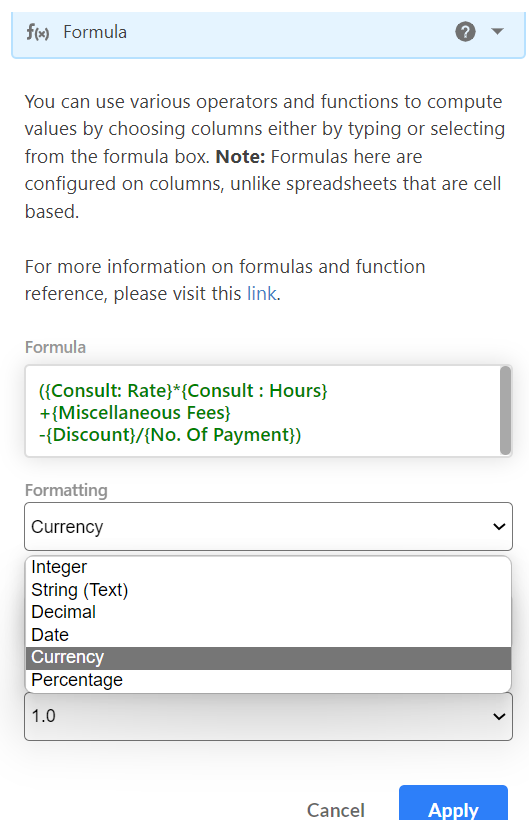In a spreadsheet, you can put a formula in any cell, and have it reference any other cell in the sheet. But Stackby is a relational database so formulas are applied for the entire column so that the same formula applies to every row in that column.

In this article, we will learn how you can do basic formula calculations

You can add multiple columns together by separating them with a + sign.

Here is the simple example of adding 3 subject marks. In the formula column you can write such
Formula:Result: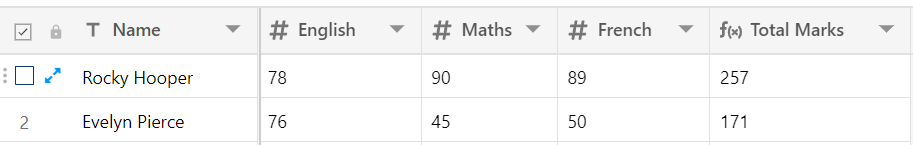2. Subtracting
You can accomplish similar calculations using the same formula structure. To find the difference between wages due and wages paid, you would use a formula like the following: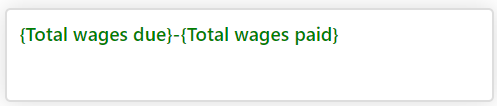Result: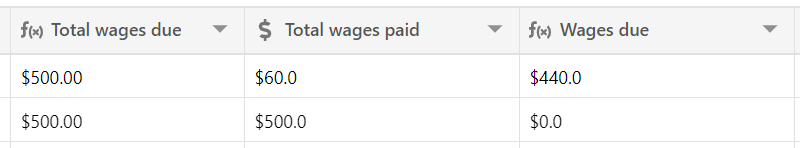3. Multiply
For example, if you want to calculate the total wages. You'll need a column hours worked, another to hours wages both are number type, and a Total wages to enter as a formula.

Formula :Result: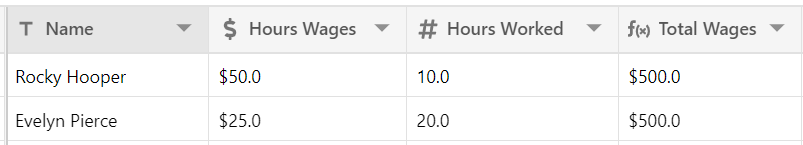4. Dividing

We can use the divide function in Stackby. Suppose if we want to find per hours wages then we can calculate it by total wages divided by No. of hours worked.

Formula:Result: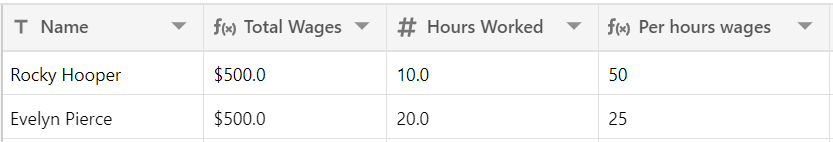5. Add, subtract, multiply, and divide in one formula

You can add, add, sub, divide within the same formula. In the below example, we can calculate the final amount to charge a client on a payment plan:

Formula:Result: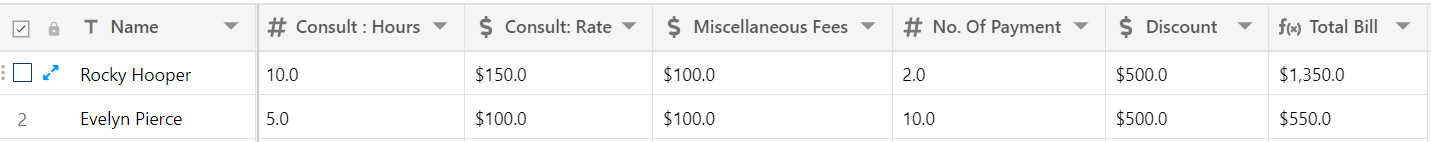6. Formatting a formula field

In the above example, if you want to do formatting then, you can apply the following formatting to the output of your formula:

Formatting in any of

• Integer

• Decimal

• Percent

• String

• Date

• Currency